Need a discount on popular programming courses? Find them here. View offers

## Data Science

Disclosure: Hackr.io is supported by its audience. When you purchase through links on our site, we may earn an affiliate commission.

# How To Use Pandas Drop Column Method For Data Cleaning

Posted in Data Science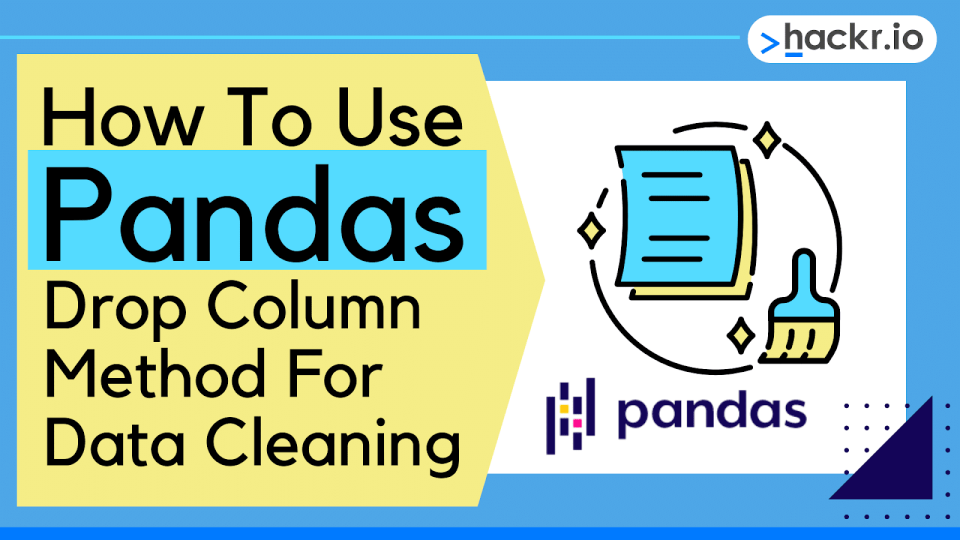Data cleaning is the process of identifying, fixing, and removing any inaccuracies or inconsistencies from a data set. It is a crucial part of most data analysis and data mining projects, and it’s usually performed before we conduct any data analysis.

Data cleaning can be a time-consuming and often tedious task, but it’s essential for providing usable data sets to data analysts and scientists. A well-cleansed dataset can be the difference between a successful data analysis project and a complete failure.

There are several ways to perform data cleaning, including the pandas drop column method. Whichever approach you take, the goal is always the same: produce high-quality, consistent data that we can use for detailed analyses.

This article will show you how to drop a column in pandas as part of the data-cleaning process.

## Why Should You Drop Columns?

Dropping columns is an important step in the data cleaning process for several reasons

• Saves time and memory: Enables faster data analysis and processing
• Improves data accuracy: Removes inaccurate, duplicate, or missing values
• Enhances data clarity: Deletes irrelevant data, i.e., if we have user information data, may not need both the first name and last name columns
• Focus on a subset of data: Removes the noise from extra columns and data
• Prevents data leakage: Restricts Machine Learning models from accessing the wrong data when predicting target variables

Pandas is a hugely popular Python library that is often used for a range of data activities including data cleaning and dropping columns from dataframes. For this reason, it’s handy to have the pandas drop column method in your toolkit, which means you need to know how it works.

Want to level up your Python & data science skills?

## Pandas Drop Column & Other Methods

We can use several methods to drop a column in pandas, whether to clean a data set or extract a dataframe with specific columns from existing data. We can also use some of these methods to make pandas drop multiple columns.

• Pandas .drop() method for rows and columns
• Pandas .dropna() method for rows and columns with null values
• Pandas .pop() for rows, columns, or individual entries
• Python del method for columns

Let’s take a look at code examples using each of these methods with pandas to remove a column from a dataframe.

## Pandas Dataframe .drop() Method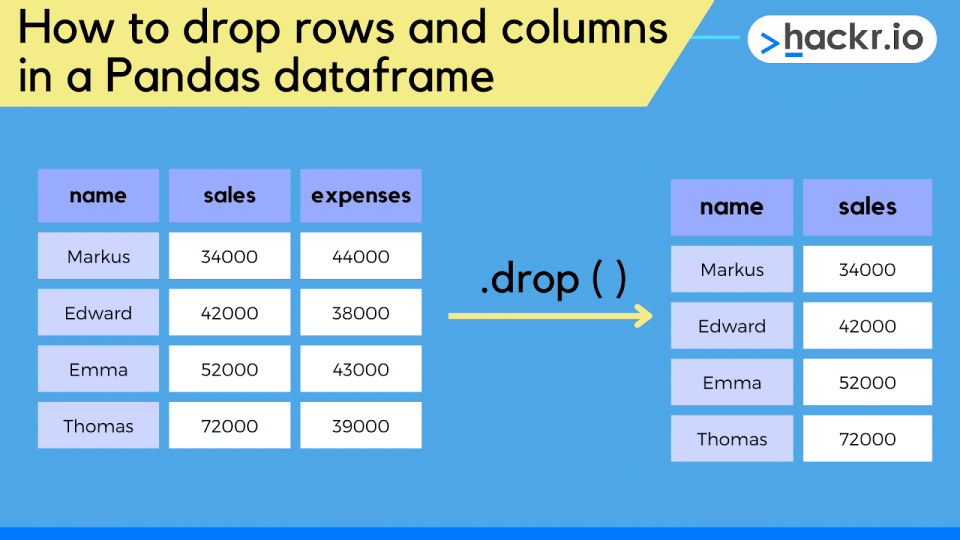We can use the .drop() method to remove rows or columns from a pandas dataframe. This is done by specifying the axis along which to drop data. By default, this will drop rows, but we can specify an axis value of 1 to drop columns instead.

The default behavior for .drop() is to return a new dataframe with the specified rows or columns removed. This means we do not modify the original dataframe (not changed inplace).

But, we can set the optional inplace argument to true to permanently remove rows or columns from our original data frame.

Note: you cannot undo an inplace drop, so check your data carefully before doing this.

We can drop rows or columns from a dataframe by either index or value. Indexes can be specified by name or number, while values require a list or a boolean mask.

Check out the official documentation if you want to learn more about the pandas .drop() method and its range of optional parameters.

Let’s jump into an example! We will load the tips.csv dataset (available for download here) into a pandas dataframe, trim this to include the top 5 rows via the .head() method, and then print it to get a feel for the data.

``````import pandas as pd

Output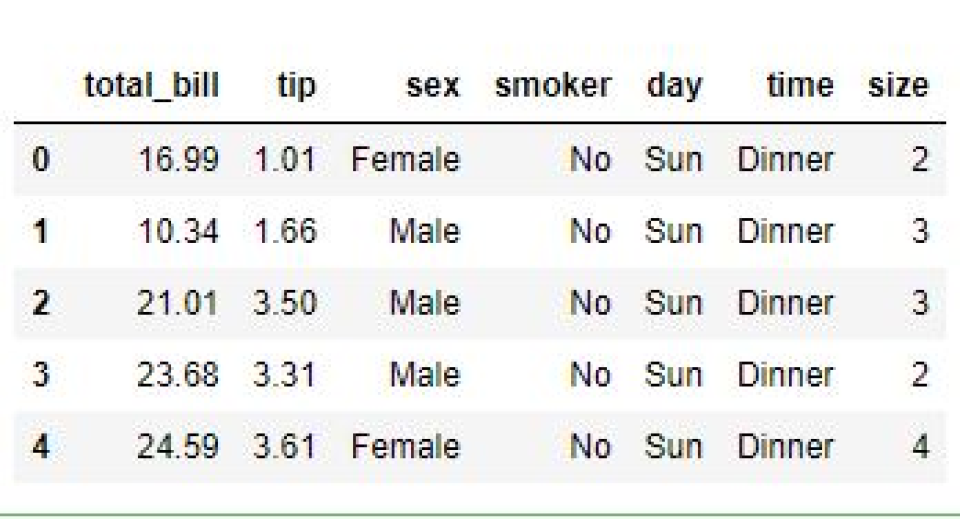### Drop Columns by Label

Now, let’s try to drop one of the columns by label (column name).

To do this, we pass the label into our .drop() call, as shown below. We also pass a value of 1 to the optional axis parameter to ensure we’re dropping columns rather than rows. If we want to drop rows, we can omit this parameter or pass a value of ‘0’.

We can then see that we’ve been able to delete the ‘tip’ column in the output.

``````df_drop = df.drop(labels='tip', axis=1)
print(df_drop)``````

Output: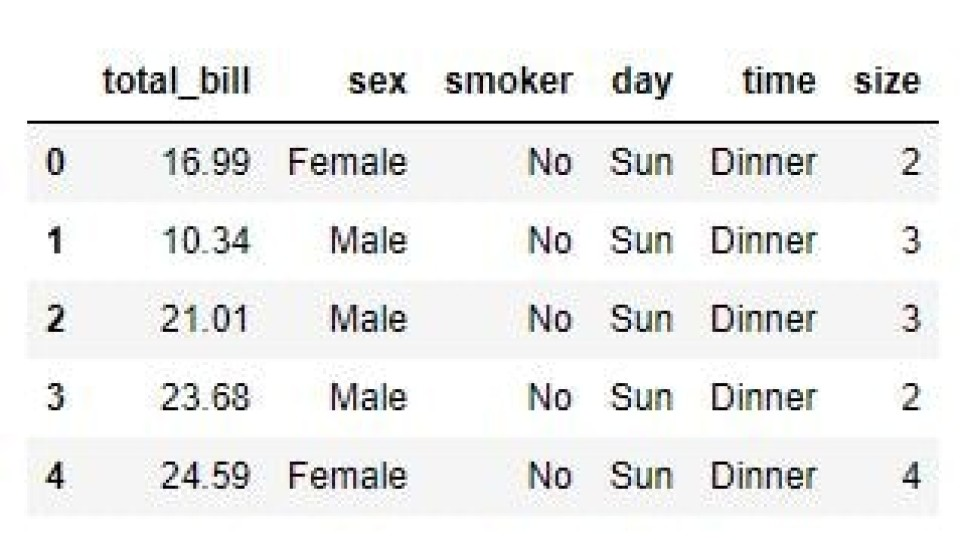If we want to drop multiple columns in pandas by label, we can pass a list of column names to the label parameter as shown below. In this instance, the resultant dataframe has lost the ‘tip’ and ‘sex’ columns, as shown in the output.

``````df_drop = df.drop(labels=['tip', 'sex'], axis=1)
print(df_drop)``````

Output: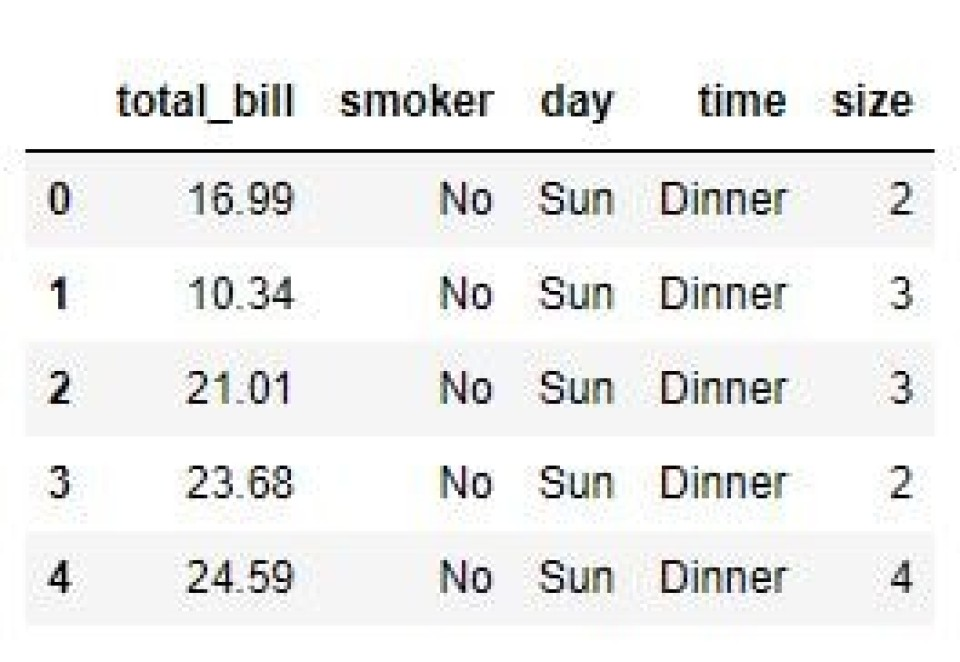### Drop Columns by Index

Another way to drop columns is with the column index. In this case, we use the index to access the column label we’d like to drop via .columns[n].

The .columns property returns a pandas index object, and we can use this to return the label for the column we’d like to drop by passing an index, n.

So, by passing an index value of 1, we can return the second column’s label (due to zero indexing). This is then used to delete the dataframe column, as shown in the output.

Note: the column with index 1 is the ‘tip’ column.

``````df_drop = df.drop(df.columns, axis=1)
print(df_drop)``````

Output: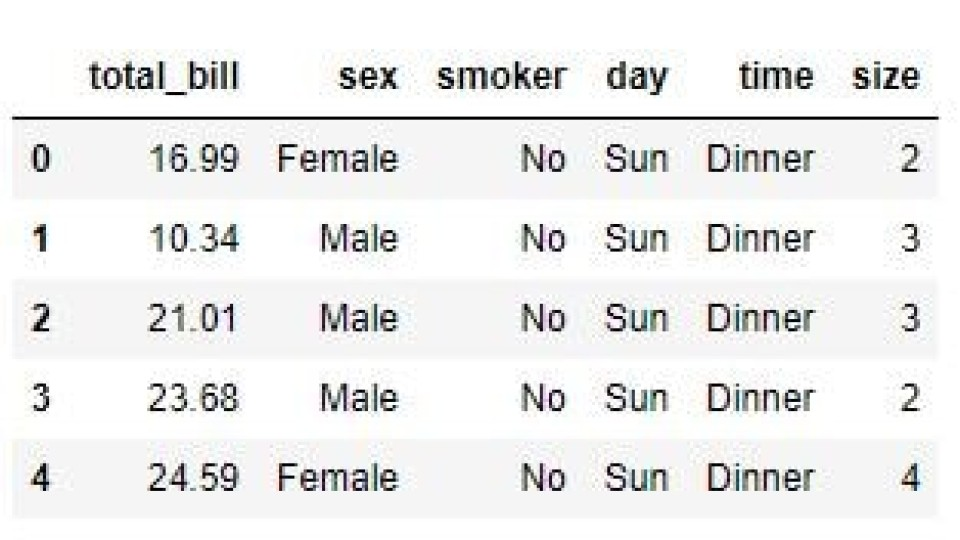In the same way that we dropped multiple columns by label, we can drop multiple columns by index. To do this, we pass a list of column index values to the pandas index object returned by .columns.

The example below shows this for indexes 1 and 2, which refer to the second and third columns. Looking at the output, we can see the resultant dataframe has lost the ‘tip’ and ‘sex’ columns.

``````df_drop = df.drop(df.columns[[1,2]], axis=1)
print(df_drop)``````

Output: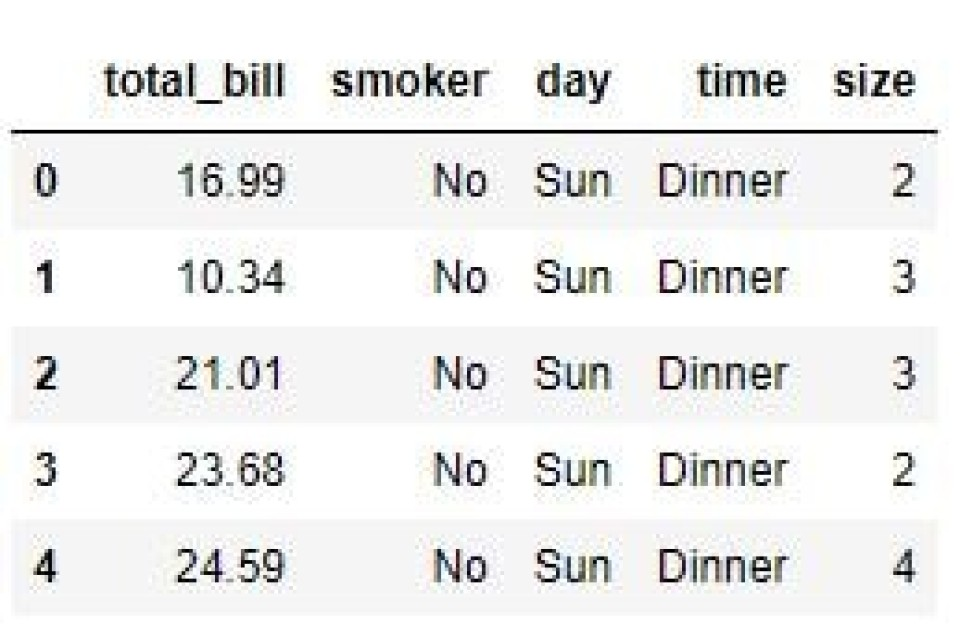### Drop Columns With the Columns Parameter

Another way to drop a column is via the optional columns parameter. In this case, we pass in the column name (just like we do with the labels parameter). And as we’ve seen already, the resultant dataframe will have dropped the ‘tip’ column.

``````df_drop = df.drop(columns='tip')
print(df_drop)``````

Output: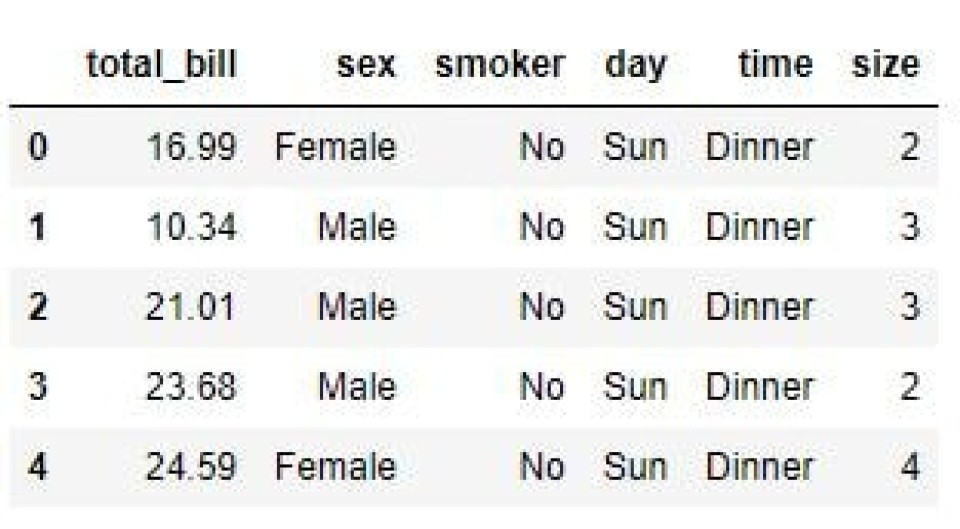We can also drop multiple columns by passing a list of column names as shown below (much like we did with the labels parameter). The resultant dataframe in the output below shows that we have deleted the ‘tip’ and ‘sex’ columns.

``````df_drop = df.drop(columns=['tip', 'sex'])
print(df_drop)``````

Output: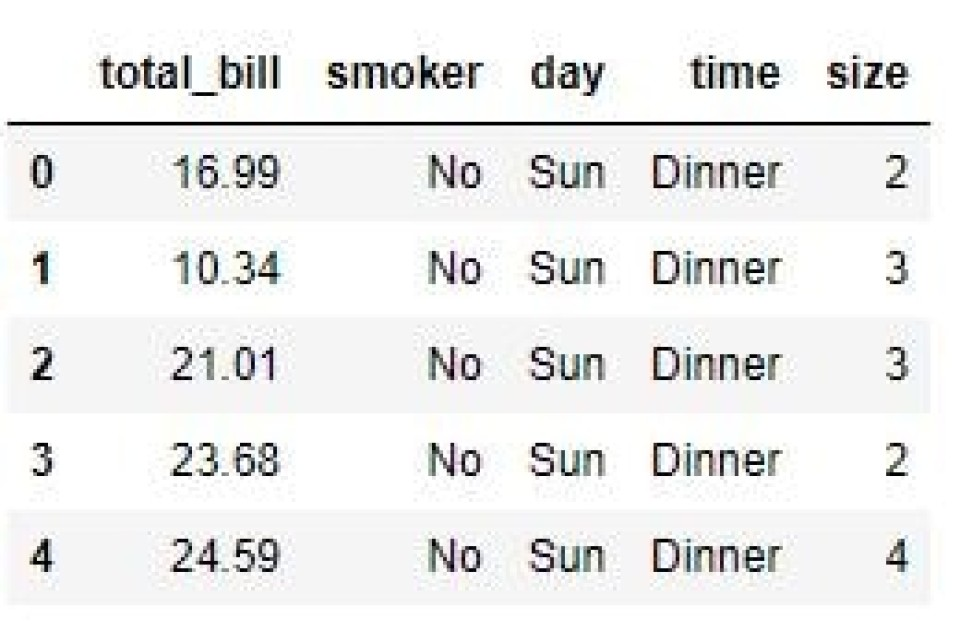### Drop Columns With DataFrame.columns.difference Method

Another useful way to drop one or more columns from a pandas dataframe is the DataFrame.columns.difference() method. This returns a complement of the argument values, which means we can use this method to drop columns from a dataframe.

For this example, let’s create a new dataframe with three columns and three rows as shown in the code snippet and output below.

``````df = pd.DataFrame([(1,2,3),(4,5,6),(7,8,9)], columns=('a','b','c'))
print(df)``````

Output: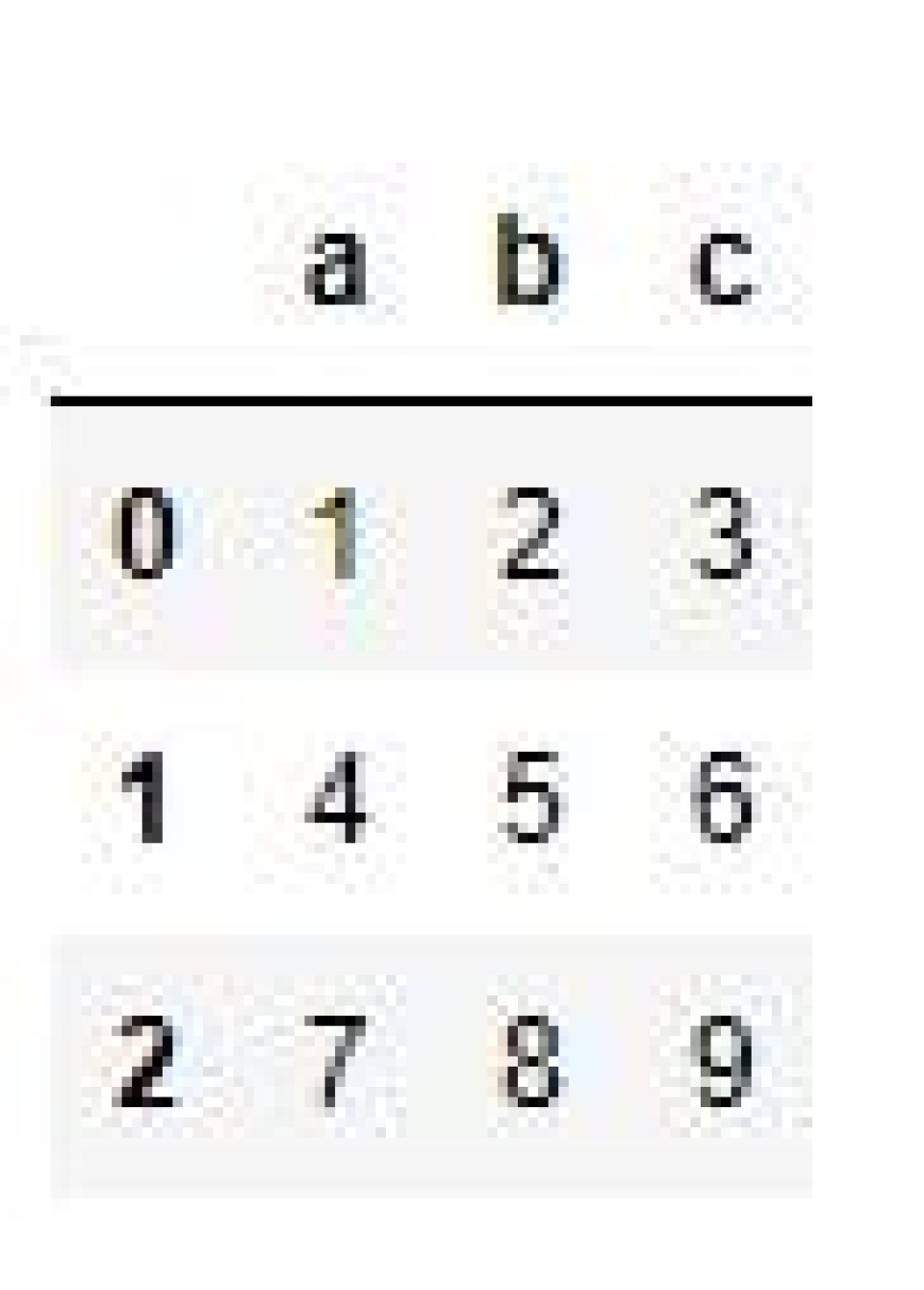If we now wanted to drop the ‘c’ column, we could use the df.columns.difference() as shown below. In this case, we pass in a list of columns we’d like to drop, which in this case, is just the label ‘c’. We can then see in the output that the resultant dataframe has lost column ‘c’.

``````df_drop = df[df.columns.difference(['c'])]
print(df_drop)​``````

Output: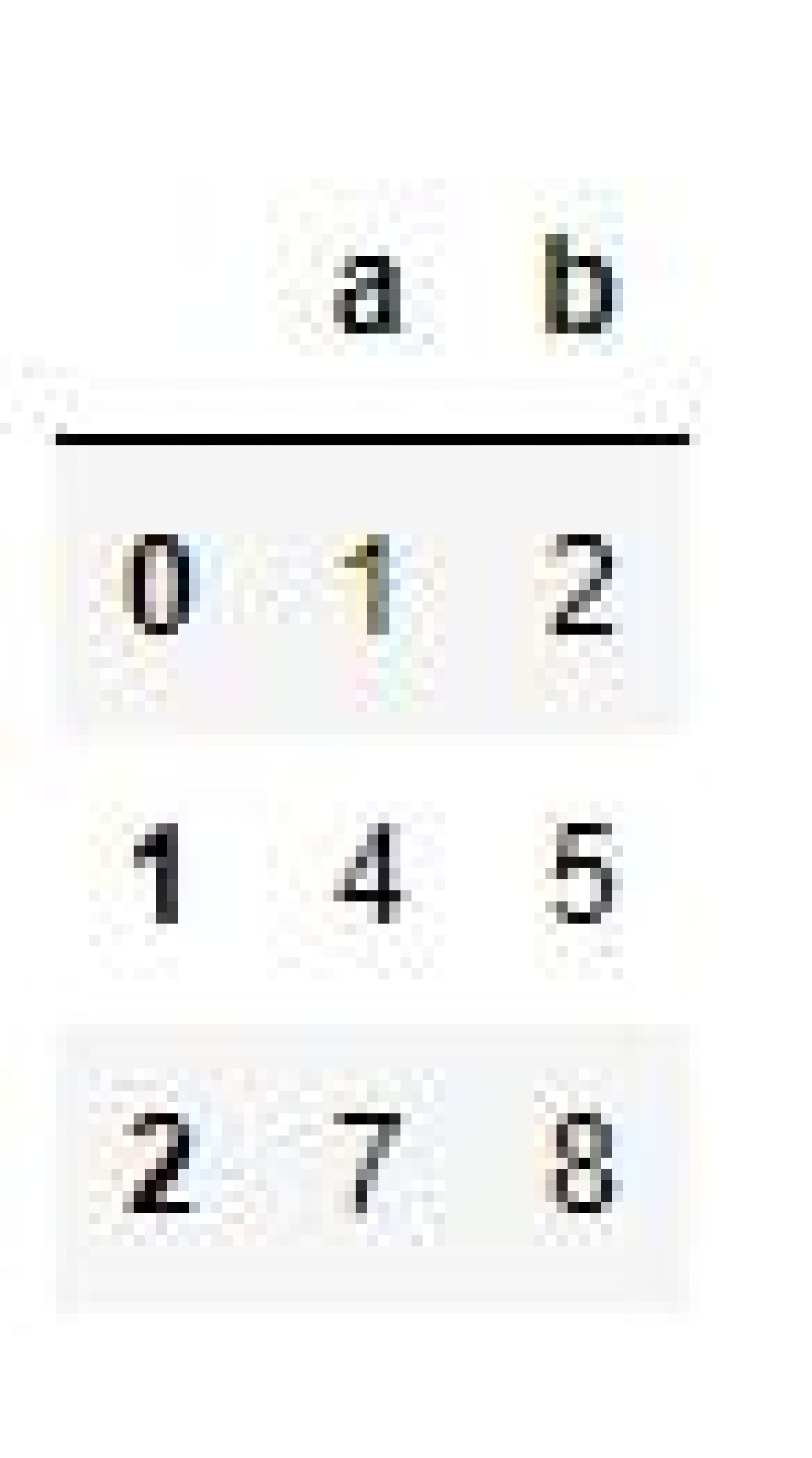### Drop Columns With .iloc() and .loc()

Another way to drop one or more columns is to use the pandas .loc() and .iloc() methods. We can use these to remove a single column, or a range of columns from a dataframe.

In the example below, we use .iloc() to delete columns 1 to 3 from the dataframe. This is because i.loc() notation is non-inclusive for the second integer argument (i.e. 4). We can see the resultant dataframe in the output, minus these the ‘tip’, ‘sex’, and ‘smoker’ columns.

``````df_drop = df.drop(df.iloc[:, 1:4], axis=1)
print(df_drop)``````

Output: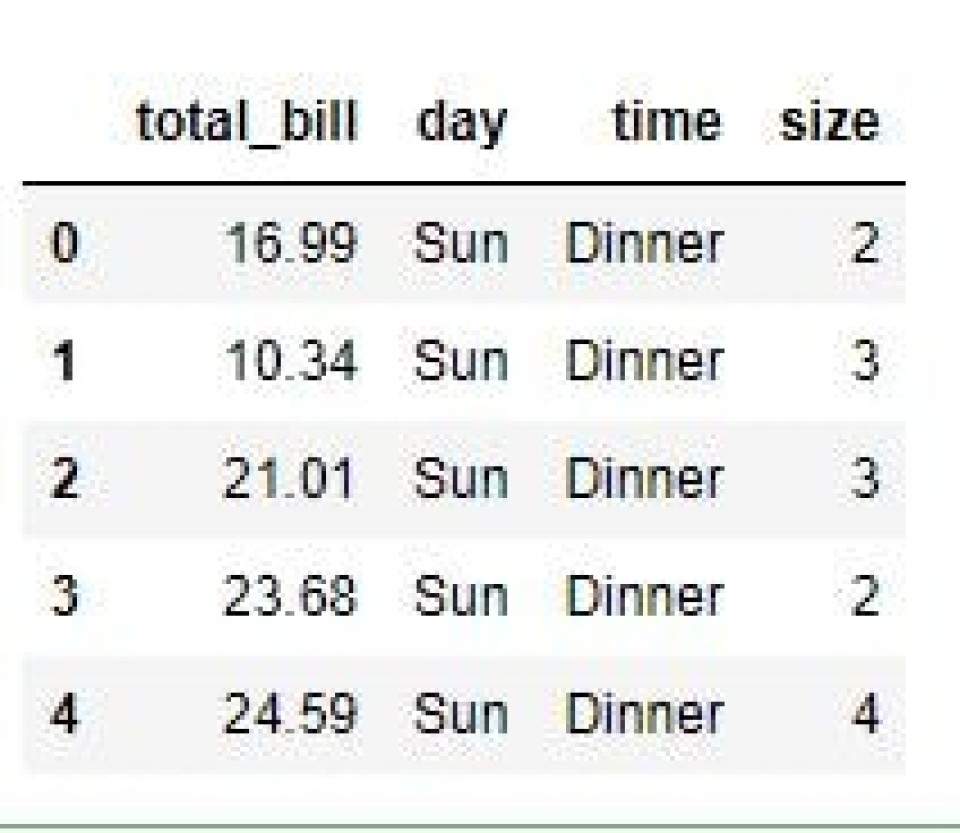Similarly, we can also use .loc() to delete columns from a dataframe, but this time we pass a list of column labels as shown below. This is because .iloc() uses integer locations and .loc() uses column labels. Looking at the output, we can see the ‘tip’ and ‘sex’ columns were dropped.

``````df_drop = df.drop(df.loc[:, ['tip', 'sex']], axis=1)
print(df_drop)``````

Output: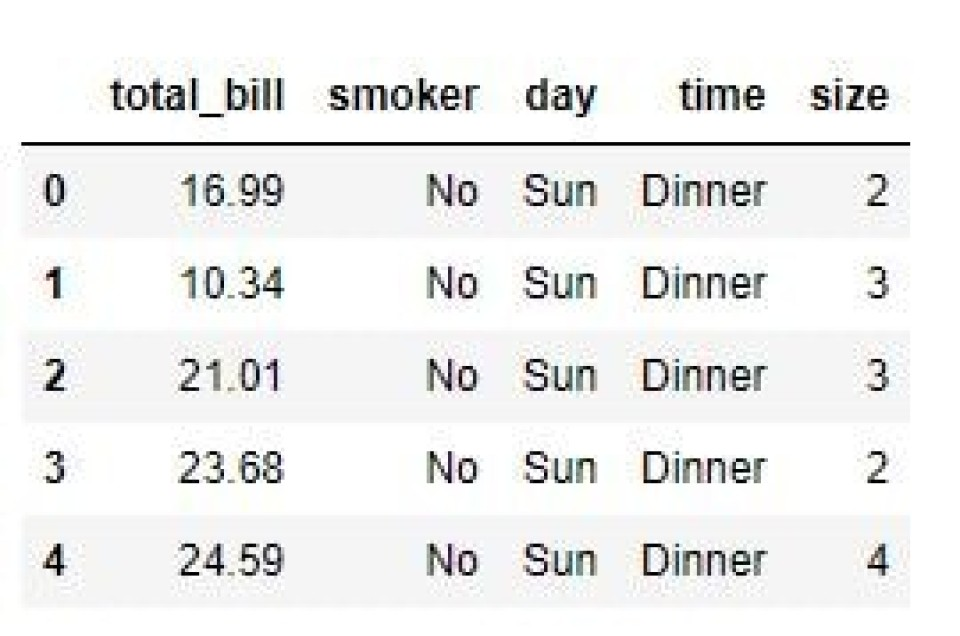### Drop Columns With a List of Labels

A quick way to delete columns with .drop() is to pass a list of column labels as the first argument.

This works because the first optional parameter for .drop() is ‘labels’, so we can omit the parameter name if we pass in a single label or a list of labels, as shown below.

The result is a dataframe minus the ‘tip’ and ‘sex’ columns, as shown in the output.

``````df_drop = df.drop(['tip', 'sex'], axis=1)
print(df_drop)``````

Output: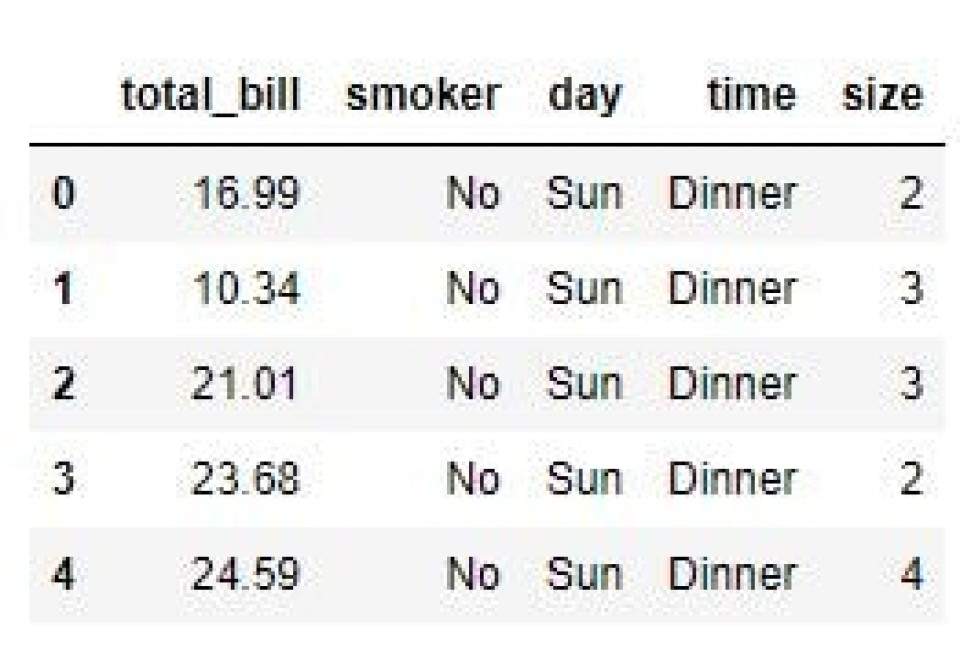## Pandas Dataframe .dropna() Method

We can use the .dropna() method to delete rows or columns from a pandas dataframe with null values. The default behavior for this method is to drop any row or column with at least one null value.

We can also specify a minimum number of null values for a row or column to be dropped by passing in a threshold argument. This can be useful if you have a data frame with lots of null values, but you only want to drop columns full of null values.

Note: this method also requires an axis argument of 1 to drop columns rather than rows.

This example will use a new dataframe with two columns and four rows. And on this occasion, we’ll use the classic Python data analysis combination of pandas and NumPy. We need to use NumPy to add ‘np.nan’ values (for null entries) to our new dataframe. Right, let’s get to it!

Looking at the output, we can see ‘NaN’ values in column ‘A’, which will be recognized as null by pandas.

``````import numpy as np
import pandas as pd

data = pd.DataFrame({"A":['1', np.nan, '2', np.nan],
"B":['3', '4', '5','6']})
print(data)​``````

Output: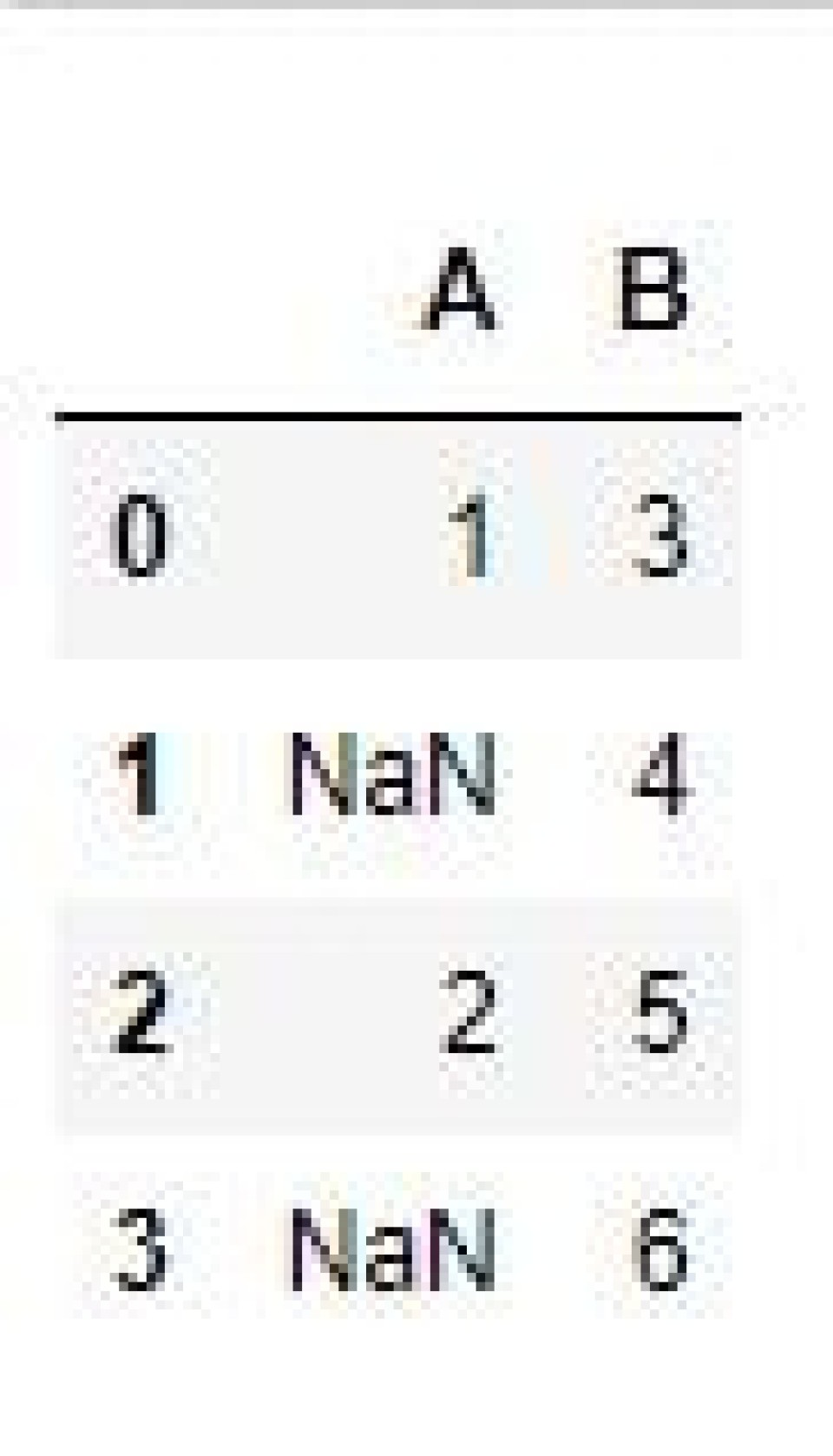Now, let’s try to drop all columns from this dataframe that have at least one null value (default behavior) with .dropna(). Looking at the output, we can see that column ‘A’ has been deleted.

``````data_drop = data.dropna(axis=1)
print(data_drop)``````

Output:Note: you can only use .dropna() to delete columns or rows with null values, it will not delete rows or columns with non-null values, for this, use .drop()

## Pandas Dataframe .pop() Method

A relatively simple way to remove a column from a pandas dataframe is to use the .pop() method. Note that this will also return the dropped column, which is fairly standard for any type of pop method.

One key difference between .pop() and .drop() or .dropna() is that it only modifies the existing dataframe inplace. This means you will be directly removing the column from the original dataframe, so please ensure you’re happy with this beforehand.

Okay, let’s create a simple dataframe with three columns and three rows as shown in the code snippet. If we then want to drop column ‘c’, we must pass the label into .pop() as shown below. The resultant dataframe in the output shows that column ‘c’ has been deleted.

Note: this method also returns the popped column. So we could save the column to a new dataframe, or work on it in another way if we wanted.

``````df = pd.DataFrame([(1,2,3),(4,5,6),(7,8,9)], columns=('a','b','c'))
df.pop('c') ​``````

Output: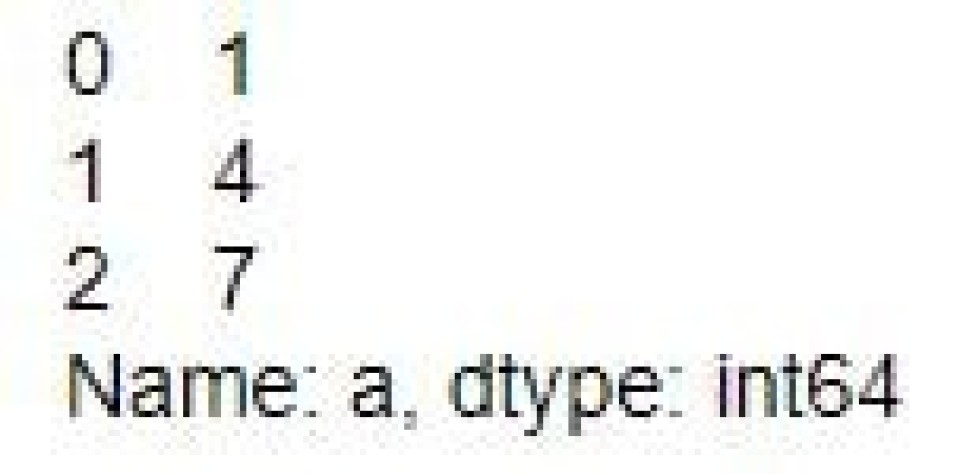As mentioned above, .pop() is a destructive method that modifies your data inplace. Because of this, it’s sometimes a good idea to make a dataframe copy beforehand. This means you can always revert if you change your mind. To do this, use the .copy() method as shown below.

``````df_copy = df.copy()
df_copy.pop('c')​``````

Some other considerations to bear in mind when using .pop()

• You’ll see an error if you try to pop a column or row that doesn't exist
• The order of any remaining columns is not guaranteed after you’ve popped a column. If you need to maintain column order, use .drop()

## Drop Columns With Python del Method

Another option for removing a column from a pandas dataframe is to use Python’s del method. Much like .pop(), this is a destructive method applied inplace to remove a column from your original dataframe.

Unlike the pandas .drop() method, you cannot use del to delete rows. Which means you can only remove columns, and these can only be deleted one at a time.

Let’s create a simple dataframe with three rows and three columns, as shown in the code and output below.

``````df = pd.DataFrame([(1,2,3),(4,5,6),(7,8,9)], columns=('a','b','c'))
print(df)​``````

Output:To drop a column, we need to access it by placing the label within square brackets. We can then pass this as an argument to the Python del method, as shown below. The resultant dataframe in the output shows that the ‘b’ column has been dropped.

``````del df['b']
print(df)
``````

Output: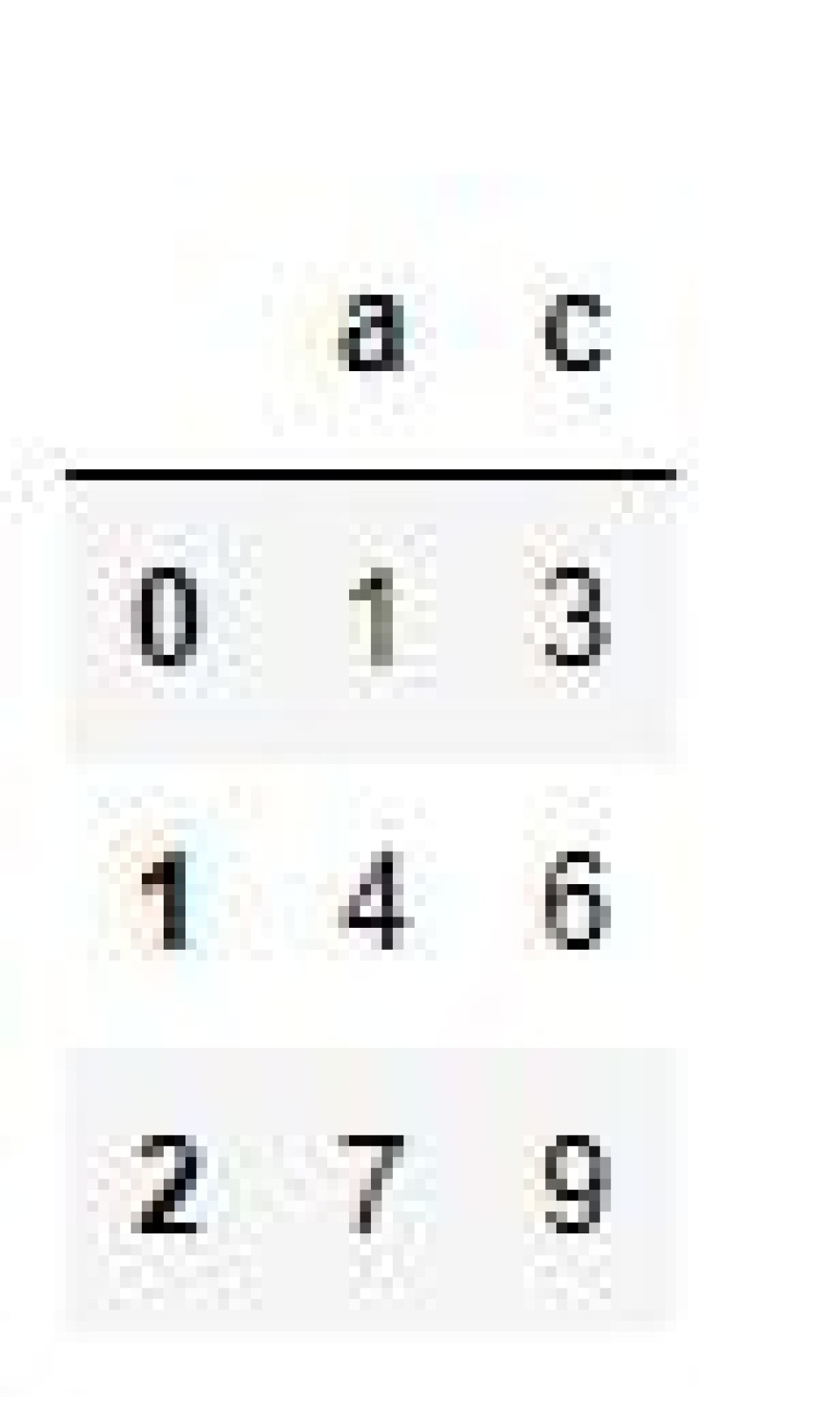Note: just like .pop(), you are unable to retrieve a deleted column after using del. If you want the option to revert, make a copy of the dataframe or use .drop() instead.

## Tips for Dropping Columns from Dataframes

1. Opt for the .drop() method to ensure your original data is retained
2. Set the axis parameter to 1 to drop columns rather than rows
3. Only set inplace to True if you want to permanently alter the original dataframe

## Conclusion

Dropping a column from a pandas dataframe is a relatively easy operation with the .drop(),.pop(), or del methods. Simply specify the column name you want to drop, and your newly reduced dataframe will be ready to use.

With that said, there are some things to bear in mind when dropping columns from a dataframe

• Ensure the column you want to drop actually exists, else you'll get an error
• If the column you’ve dropped is being used in any calculations or operations, these will need to be updated to reflect this change
• If you have any other dataframe dependencies, these will need to be updated to reflect the change

And that’s it! The pandas drop column operation in a dataframe is a simple, but potentially destructive action. So make sure you know what you're doing before you drop a column!

Want to boost your Python skills? Check out:

#### 1. How Do I Drop a Column by Index?

To drop a column by index, use the following syntax

``df.drop(df.columns[index], axis=1)​``

#### 2. How Do I Drop a Column With No Name?

We can’t drop a column with no name.

#### 3. How Do I Drop Multiple Columns in Pandas?

To drop multiple columns in pandas, you can use the .drop() function. For example, to drop the columns 'a' and 'b', you would do the following

``df.drop(['a', 'b'], axis=1)​``